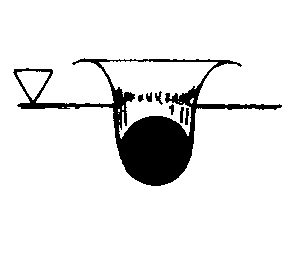# Electric Boat Corporation: Underwater Express

 Since July 2009 it is known, that the Electric Boat Corporation (subsidiary company of General Dynamics) by order of the US Government develops a test-submarine (length ca. 30 m) that is planed to reach by means of supercavitation running velocities of 100 knots (ca. 185 km/h). The project is named "Underwater Express". It is intendet to deliver men and material faster. In spring 2010 the test runs of the boat should be finished. But until Decembre 2011 more information were not available. Now I will try to analize the problems of a supercavitating submarine and calculate an example presuming certain assumptions. Dimensions and Shape. Assumptions: Length = 30 m, diameter = 3 m , shape: nearly rotational ellipsoid. The length/diameter-ratio is 10:1. Assuming a running depth of 10 m and using the length/diameter-ratio delivers a cavitation number   σ = 0,036 (s. chapter Cavitation Laws). During the supercavitational running this cavitation number has to be kept constant. Conventional Underwater Running. A submarine shaped like a cavitation bubble has the smallest friction compared with any other shape because flow velocity is constant on the whole surface. The estimated resistance at a velocity of 30 knots ( ca. 46,5 km/h) should be in the region of 60 - 80 kN. For supercavitational running the nose region of the vessel has to be changed. The simplest way to do so is cutting the nose region vertically to the axis forming a flat bow with a diameter of 0.63 m. This increases the resistance during normal running of 30 knots estimated to be 100 kN affording a power of 1500 kW. Pressurized air ejection for bubble formation The purpose of air ejection is generation of an air space around the submarine. For reducing air consumption the layer should be as thin as possible because the transport volume of air reduces the distance of supercavitational running. It is assumed that a constant flow of air through many small holes in the front region of the submarine may produce a layer of constant thickness. In the rear region air must be extracted to reduce thickness of air layer and to reduce air consumption. The ajustment of an air layer around the submarine seems a difficult but solvable technical problem. Supercavitational running. Resistance of a flat plate of 0.63 m at velocity of 100 knots is ca. 350 kN. It is assumed that ca. 70% of the momentum required to produce the cavity can be recovered at the heck where the collapsing water delivers a momentum in direction of the motion (see chap. Hydrodynamic Paradox). In this case the resistance of the submarine in normal running at velocity of 30 knots equals the resistance while supercavitational running at velocity of 100 knots. It seems possible that even higher momentum recovery is possible so that higher velocities are accessible with the same power.
home                                               back                                               more